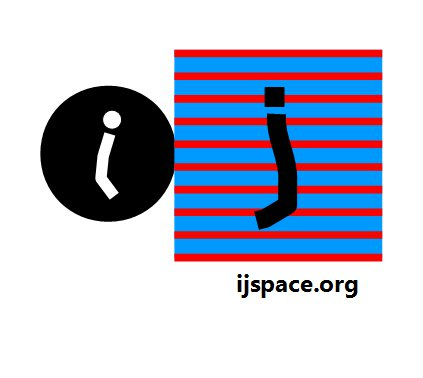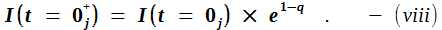Home

Summary: The value of the fine structure constant Alpha in low energy limit and its significance based on the discrete measurement space, is discussed.We have so far described the system in terms of measurements.  A measurement has two components, (i) an application of the measurement force and (ii) the measurement of response.  Both these components combined together are called an interaction.  An interaction and a measurement are analogous to each other.  We make a simple hypothesis that,

" Every observer making a measurement of the environment or a system, is simultaneously measured by the environment or the system."

Above statement is a generalization of the the Newton's third law of equal and opposite reaction.  It differs from the classical definition in the sense that the object interacting with each other, are of different capabilities.  Therefore the measurement forces applied by the objects in j-space will be different and may not be measurable by the objects interacting with each other.  We can also say that the object with significantly lower capability may not know that it is being measured.
In classical mechanics both objects have equal capabilities and hence equal and opposite reactions.  An equivalent statement can also be made that an observer in the discrete measurement space can not make a measurement without being measured.

In an atomic structure the electrons interact with each other with photons.  Which is equivalent to saying that the electrons measure and are measured with photons.  Further the wave-particle duality allows the photons to be represented by the electromagnetic waves.  The electromagnetic waves have the vectors E(electric vector) and H(magnetic vector) representing a relationship, which is progressing with time and defined classically by Maxwell's equations.  The vectors E and H are considered to be at a phase difference of π÷2 in the physical space.

We also derived a relationship for the progress of the information in the discrete measurement space or  j-space as,Above relationship describes how a continuous information source is measured in discrete j-space by an observer of finite capability.  Thus in j-space we have a relationship describing the variation by a factor e1-q which was derived without any physical considerations of the space.  And we also have another relationship which provides a phase π÷2 between the force and response in physical space.

In other words we have two dimensionless variables e1-q and π÷2 which characterize the progress of the information I(t = 0j), on the time axis in j-space and the measurements made by Obsj in j-space using EM based measurement tools respectively.  We take the ratio of both to compare.  The values of term [e1-q÷(π÷2)] for some of the initial q-values are shown below:

 q X = e1-q Y = [X ÷ (π÷2)] α = Y2 1÷Y2 Comments 1 1 6.366 x 10-1 5.053 x 10-1 2.467 event in j-space 2 3.679 x 10-1 2.342 x 10-1 5.485 x 10-2 1.823 x 101 Elementary structures in j-space (yet to be measured, not enough resources) 3 1.353 x 10-1 8.616 x 10-2 (.08542455) 7.423 x 10-3 1.347 x 102 (137.04) Atomic structures in j-space 4 4.979 x 10-2 3.170 x 10-2 1.005 x 10-3 9.954 x 102 Values related to macroscopic structures in j-space. The value of q can increase up to  ∞j-. 5 1.832 x 10-2 1.166 x 10-2 1.360 x 10-4 7.355 x 103 6 6.738 x 10-3 4.290 x 10-3 1.840 x 10-5 5.435 x 104 7 2.479 x 10-3 1.578 x 10-3 2.490 x 10-6 4.016 x 105 8 9.119 x 10-4 5.805 x 10-4 3.370 x 10-7 2.967 x 106

Let us consider the value of Y for q = 3, it is 8.616 x 10-2.  The value is calculated based on assumption that the angle between E and H is π÷2.  The corresponding value of 1÷Y2 is 134.7.  The standard value for 1÷Y2 is approximately 137.04.  The exact value of the fine-structure constant corresponds to q ~ 3.009.  We have to keep in mind that q is calculated based on the ratio of two irrational functions hence can not be an exact natural number.  The known standard values are shown in parenthesis.  The number 0.8542455 for the variable Y represents the amplitude for an electron to emit or absorb a photon, also known as the coupling constant.  We square this number to get the fine-structure constant alpha (1÷137.04).

The relationship [e1-q÷(π÷2)], does not require any prior knowledge of a physical structure and it does not use any measured physical constants such as electric charge e, speed of light c or the Planck's constant h in its derivation.  This relationship should be applicable to stable structures in discrete j-space, which are measured using electromagnetic interaction with the specific structure.  Thus the introduction of the value of π÷2 provides results for the phenomena in which the measurement using electron-photon interaction, plays an important role.

We also note that the values calculated using the factor e1-q, represent the values in the asymptotic region as each q value represent a PE1 event.  For phenomena where interactions between the structure being measured and the measurement signal, are of nature other than EM, appropriate dimensionless constant(s) need to be introduced in place of π÷2.

We have described the nature of the system based on PE1 measurements.  These measurements do not assume a priori existence of variables or any known relativistic or non-relativistic coordinate systems.  Only condition placed is the requirement of PE1 events.  The description provided by the observer is based on the measurements only and the variables or reference systems must be derived from the information obtained from these measurements.  The description of the system using the Cartesian coordinates or space-time coordinates will be required when the application of the measurement force is necessary to make an observation, and correlation needs to be made with experimental results.

What we are suggesting, is that the physical structures measured are the components of the information space represented by (e1-q), whereas the observer's measurement capability (π÷2) is represented by the phase relationship between E and H.  The fine-structure constant under the low-energy limit tells us where do we stand in terms of measuring an infinite information space in a discrete measurement space.   We take note that we are essentially measuring δi in j-space.

The term "fine-structure constant" represents electron-photon coupling in zero-energy limits.  It is known that the value of alpha approaches the value 1÷128 for the interaction energies above 80GeV.  Thus alpha is not really a constant but dependent on the interaction energy.  The relationship of alpha with q-values also shows it, as the interaction energy would be higher for low-q values and therefore the value of alpha would go up with increasing interaction.

It is often asked if alpha is not really constant then why worry about explaining its value?  We need to remember that the value 1÷137 still represents the one end of the spectrum, the end at which we the macroscopic observers (ObsM), exist and measure.  Therefore it is important to understand the value of alpha at the low energy limit. If the interaction energies are increased further the value of alpha would go up then what does that mean in terms of q-values?  The value 80GeV corresponds to Δq equal to approximately .068.  The ionization potential of 13.6eV corresponds to Δq equal to approximately 1.16×10-10.  Our playing ground is fairly small in q-space.

We may also want to think, if the atomic structure is q = 3 state, then what is the significance of q = 2 state?  Admittedly it represents more information than available for q = 3 state.  It will be most interesting to find out or even be able to predict what type of structures would we observe, if we could measure q = 2 state.  One thing for sure that there is a huge amount of energy involved.  And then what do the value of q higher than 3 represent? Surely these structures are easily measurable or in other words they should be right in front of us.

Also if we can not measure the origin accurately, how can we be so sure that the vectors E and H are at exact 90o angle to each other? How can we correlate this specific issue of angle between E and H being exactly 90o to the restriction of the 3-dimensional space? We will attempt to answer this question next." I believe that it will only be possible to write the conclusion if a theory will be established which will determine the value of the fine-structure constant and will thus explain the atomistic structure of electricity, which is such an essential quality of all atomic sources of electric fields actually occurring in Nature."

- Wolfgang Pauli, "Exclusion principle and quantum mechanics", Nobel Lecture, December 13, 1946.

"It has been a mystery ever since it was discovered more than fifty years ago, and all good theoretical physicists put this number up on their wall and worry about it."

- Feynman writing about the fine-structure constant in "QED: The strange theory of light and matter", Princeton University Press.

Bethe, Hans A. and Salpeter, Edwin E., “Quantum Mechanics of One- and Two-electron Atoms”, Springer Verlag and Academic Press, 1957, is a good reference.

The correlation between the electromagnetic mass, rest mass and the fine structure constant is discussed in Brillouin, L., “Science and Information Theory”, Academic Press, New York, 1962